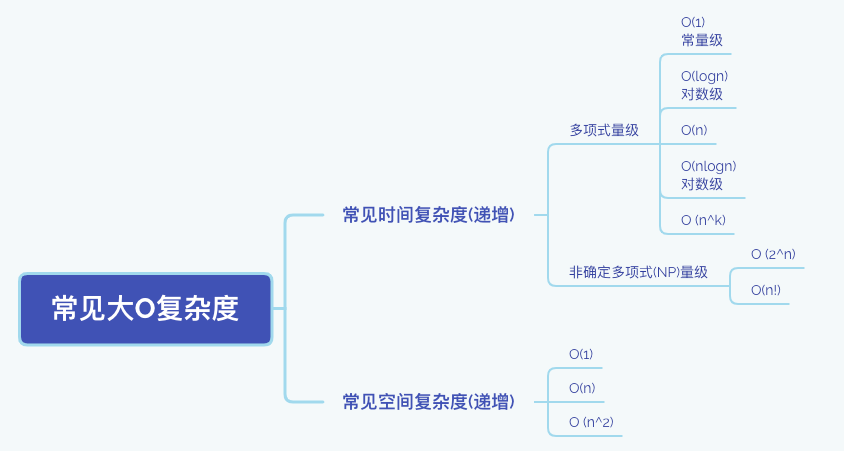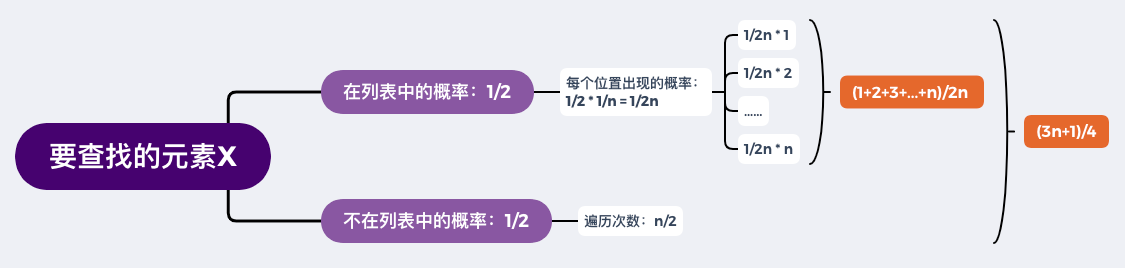# 算法复杂度分析¶

• 快：执行效率，即时间复杂度
• 省：资源消耗，即空间复杂度

## 动态分析法¶

• `time`
``````import time

start_time = time.time()  # 开始时间
pass  # 待测算法
end_time = time.time()  # 结束时间

print(end_time - start_time)  # 耗时
``````
• `timeit`
``````timeit.timeit(stmt, setup,timer, number)
"""
stmt: statement的缩写，要测试的代码或者语句，默认值是"pass"
setup: 在运行stmt前的配置语句，默认值"pass"
timer: 计时器，一般忽略这个参数
number: stmt执行的次数，默认是100万次
"""
# 由于stmt只能是语句，需要借助匿名函数来测试函数
t = timeit.timeit(lambda :range(10), number=500)
print(t)
``````

## 静态分析法¶

• 每行代码执行时间：`t`
• 数据规模：`n`
• 代码总执行次数：`f(n)`
• 代码总执行时间：`T(n)=f(n)*t`

### 分析法则¶

• 找循环次数最多的代码段
``````def test():
sum = 0  # t
i = 1  # t
while i <= n:  # nt
sum = sum + i  # nt
i = i + 1  # nt

return sum

# T(n) = (2+3n)t
# 复杂度：O(n)
``````
• 找嵌套最多，量级最大的那一段代码
``````def test():
sum = 0  # t
i = 1  # t
while i <= n:  # nt
j = 1  # nt
while j <= n:  # n^2t
sum = sum + i * j  # n^2t
j = j + 1  # n^2t

return sum

# T(n) = (2+2n+3n^2)t
# 复杂度：O(n^2)
``````
• 若最大有多段则相加，有嵌套则相乘
``````def f1(n):
ret = 0
i = 1
while i < n:
ret = ret + f2(i)  # 这里调用了另一个函数f2
n = n + 1

def f2(n):
sum = 0
i = 1
while i < n:
sum = sum + i
return sum

# T(n) = T1(n) * T2(n)
# 复杂度为：O(n^2)
``````## 时间复杂度分析¶

• 常量级

``````i = 8
j = 6
sum = i + j
``````
• 对数级

`````` i=1
while i <= n:
i = i * 2

# 退出循环的条件为2^x=n，即要循环x=log2_n次
# 忽略底数之后，复杂度为：O(logn)
``````

### 特殊情况¶

``````# 示例1
# 在一个长度不固定的无序列表中，查找元素x所在的位置
# 找到则停止查找，遍历完后没找到则返回-1
def find(_list, x):
pos = -1
for i in len(_list):
if _list[i] == x:
pos = i
break
return pos
``````

• 如果x位于首位则时间复杂度为O(1)，称之为`最好情况时间复杂度`
• 如果x位于末位，则时间复杂度为O(n)，称之为`最坏情况时间复杂度`
• 但大多数情况下元素都不位于首位或末位，于是我们需要引出`平均情况时间复杂度```````# 示例2
# 向一个长度为n的列表中插入新数据value，如果列表有剩余空间，则直接插入
# 如果列表没有剩余空间，则先计算其元素总和sum，然后清空列表后将sum插入到列表的首位，再继续插入value
_list = [1,2,3...n]
count = 0
def insert(value):
if count == len(_list):
sum = 0
for i in len(_list):
sum = sum + _list[i]

_list.insert(0, sum)
count = 1

_list.insert(count, value)
count = count + 1
``````

• 理想的情况下，列表有剩余空间，直接插入即可，即最好情况时间复杂度为O(1)
• 最坏的情况下，没有剩余空间，需要遍历一次列表求和，所以最坏情况时间复杂度为O(n)
• 平均情况呢？此处与示例1有所不同